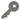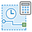# Calculate Motion StatisticsAvailable in real-time and big data analytics.

The Calculate Motion Statistics toolcalculates motion statistics and measures for event features based on Track ID and Start Time key fields. Calculations include distance, time span, height, speed, acceleration, heading, and idling based on the provided time values and geometry of point-based events.

## Example

A city is monitoring snowplow operations and city officials want to better understand vehicle movement. The Calculate Motion Statistics tool can be used to determine idle locations and time spent idling, average and maximum speeds, and total distance covered, as well as other statistics.

## Usage notes

• The Calculate Motion Statistics tool returns all input observations; therefore, the first observation for each track will have null values for motion statistics. Until there are enough observations in the track to satisfy the history depth, the first few observations will calculate motion statistics using available observations. Once there are enough features to satisfy the history depth, motion statistics will be calculated using the number of features indicated by the history depth.
• Idling is determined based on whether an observation has moved less than the distance tolerance and the time between observations is greater than the time span tolerance.
• The units of the output motion statistics depend on the units specified for the Distance Tolerance and Timespan Tolerance parameters. For example, if you're specifying a Distance Tolerance value of 750 meters and a Timespan Tolerance value of 10 minutes, the output distance fields will be in meters, the output time span fields will be in minutes, and the output speed fields will contain values with units of meters per minute.
• The Method parameter specifies how distances and other spatial calculations are made. Options include the following:
• Planar—Euclidean calculations will be generated. Euclidean distance is measured in a two-dimensional Cartesian plane in which a straight-line is calculated between two points on a flat surface (the Cartesian plane). Euclidean distance is the more common type of distance calculation and works well when analyzing distances around features in a projected coordinate system that are concentrated in a relatively small area such as one UTM zone. Euclidean distances are referred to as planar distances.
• Geodesic—Geodesic calculations will be generated. Geodesic distances account for the actual shape of the earth (an ellipsoid, or more properly, a geoid). Distances are calculated between two points on a curved surface (the geoid) as opposed to two points on a flat surface (the Cartesian plane). Consider using geodesic calculations in the following circumstances:
• The input features are dispersed (cover multiple UTM zones, large regions, or the entire globe).
• The spatial reference (map projection) of the input features distorts distances to preserve other properties such as area.
• In a real-time analytic, the tool operates in a stateful manner, allowing it to compare sequential observations to one another to calculate motion statistics—for example, how the current position, speed, altitude, and other statistics are different from that of the previous observation.
• The Target Time Window parameter in a real-time analytic should be at least as long as the longest anticipated interval between observations for any given track. Observations older than this duration will be removed from memory to manage resources
• In real-time analytics, the Calculate Motion Statistics tool maintains a state store of the current observation for each Track ID. When a new observation is received it is compared to the current observation of the same Track ID, if present, to calculate motion attributes. If the new observation has a newer timestamp than the current observation in the state store, the current observation is replaced. If it has an identical timestamp to the current observation for the same Track ID, the tool does not distinguish between the two observations. This means the tool will not replace the current observation in the state store with the new observation, the new observation will not be added to the state store and future observations for this Track ID will be compared to the current observation that remained in the state store until a new observation arrives with a later timestamp. A warning will be logged by the tool when this occurs.
• In big data analytics, one or more fields can be specified to identify tracks in addition to the Track ID field. Tracks are represented by the unique combination of one or more track fields.

## Parameters

ParameterDescriptionData type

Input Layer

The point features for which density will be calculated.

This dataset must have Track ID and Start Time key fields defined.

Features

Timespan Tolerance

The time span tolerance value that will be used to determine idling.

Idling is determined based on whether an observation has moved less than the distance tolerance and the time between observations is greater than the time span tolerance.

String

Distance Tolerance

The distance tolerance value that will be used to determine idling.

Idling is determined based on whether an observation has moved less than the distance tolerance and the time between observations is greater than the time span tolerance.

String

History Depth

The number of event observations to evaluate per track (includes the current observation). Motion calculations will be done based on this information.

For example, if you set a History Depth value of 3, each time a record is processed, the motion calculations such as average speed will calculate this value based on the speed of the current observation and the previous two observations.

##### Note:

The first few observation for each track will have null values for motion statistics. The next observations while still fewer than the history depth will have motion statistics calculated based on existing features that are less than the history depth. For example, if you set the History Depth value to 3, the first features for each Track ID feature will have null motion statistics. The second and third features for each Track ID will have motion statistics calculated from the available features.

Integer

Method

Specifies whether the distance calculation method will be Geodesic or Planar (Euclidean).

##### Note:

If Planar is chosen, the input points must be projected. You can use the Project tool to project the input features before calculating distances.

String; Allowed values: Planar | Geodesic

Target Time Window

The longest anticipated period of time between observations for each track. This applies to real-time analytics only.

String

Track ID Fields (big data analytics only)

The fields that uniquely identify features to be processed. The parameter will be automatically populated if a Track ID key field is set on the Input Layer value.

Fields

## Output layer

The output layer will contain the attributes described below appended to the original attributes for each feature. All statistical attributes (min, max, and so on) are calculated based on the specified History Depth value. The first few observations for each track will have null values for most motion statistics until there are enough observations in the track to satisfy the history depth. For example, if you set the History Depth value to 3, the first three features for each Track ID feature will have null motion statistics.

##### Note:

The units of the output motion statistics depend on the units specified in the Distance Tolerance and Timespan Tolerance parameters. For example, if you're specifying a Distance Tolerance value of 750 meters and a Timespan Tolerance value of 10 minutes, the output distance fields will be in meters, the output time span fields will be in minutes, and the output speed fields will contain values with units of meters per minute.

Field nameDescriptionField type

Distance

Distance traveled since the previous observation

Float64

TotalDistance

Total distance traveled

Float64

MinDistance

Minimum distance traveled

Float64

MaxDistance

Maximum distance traveled

Float64

AvgDistance

Average distance traveled between observations

Float64

Speed

Current speed since the previous observation

Float64

CumSpeed

Cumulative speed

Float64

MinSpeed

Minimum speed

Float64

MaxSpeed

Maximum speed

Float64

AvgSpeed

Average speed

Float64

TimeSpan

Elapsed time since the previous observation

Float64

TotalTimeSpan

Total elapsed time

Float64

MinTimeSpan

Minimum elapsed time

Float64

MaxTimeSpan

Maximum elapsed time

Float64

AvgTimeSpan

Average time span

Float64

CurrentHeight

Current height of the observation

Float64

ChangeHeight

Change in height from the previous observation

Float64

TotalChangeHeight

Total change in height from the first observation in the history depth to the current observation

##### Note:

This can be a negative value.

Float64

MinHeight

Minimum height observed

Float64

MaxHeight

Maximum height observed

Float64

AvgHeight

Average (mean) height observed

Float64

Slope

Current slope of travel since the previous observation

MinSlope

Minimum slope

Float64

MaxSlope

Maximum slope

Float64

AvgSlope

Average slope

##### Note:

Average slope is calculated based on the slopes observed since the beginning of the history depth. If the history depth is 3, average slope is the average of the slope from observation 1 to observation 2 and from observation 2 to observation 3.

Float64

Acceleration

Acceleration since the previous observation

Float64

TotalAcceleration

Total acceleration

Float64

MinAcceleration

Minimum acceleration

Float64

MaxAcceleration

Maximum acceleration

Float64

AvgAcceleration

Average acceleration

##### Note:

Average acceleration is calculated based on the accelerations observed since the beginning of the history depth. If the history depth is 3, average acceleration is the average of the acceleration from observation 1 to observation 2 and from observation 2 to observation 3.

Float64

Idling

Specifies whether the track is idle based on a distance and time threshold from the previous observation

Boolean

TotalIdleTime

Cumulative amount of time for which idling was detected

Float64

PercentageIdleTime

Percentage of time for which idling was detected

Float64